(1) 根式解问题；

(2) 根的分布及近似计算；

(3) 根的存在问题；

(4) 根的性质的研究.

§1   二、三、四次方程的根的表达式

1. 基本概念

[数域如果一个数系满足下列两个条件，则称这个数系为一个数域：

(i) 系中有不等于零的数；

(ii) 对系内任意两个数（这两个数也可相同）的和、差、积、商（零不能作除数）仍为系内的数，这就是说，系内的数对于四则运算是封闭的.

例如，有理数系、实数系、复数系都是数域.

[多项式的根形如

f(x)=a0xn+a1xn-1+a2xn-2+L+an-1x+an=0

c是一个常数,使f(c)=0,则称c为多项式f(x)或方程f(x)=0的根.本节先考虑在实数域上的二、三、四次方程.

2. 二次方程

 方程 ax2+bx+c=0 x2+px+q=0 根的表达式 x1,2=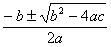x1,2=根与系数的关系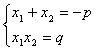判别式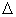=b2－4ac>0 有两个不等的实根=0 有两个相等的实根<0 有两个复根=p2－4q>0 有两个不等的实根=0 有两个相等的实根<0 有两个复根

3.三次方程

[x31=0]  方程                   x31=0

[x3+px+q=0(卡尔丹公式)]   方程

x3+px+q=0

x1=x2=ω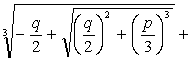ω2(2)

x3=ω2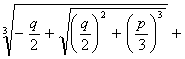ω根与系数的关系为

x1+x2+x3=0,,   x1x2x3=q

判别式为=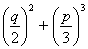>0时，有一个实根和两个复根；=0时，有三个实根，当p=q=0时，有一个三重零根；当时，三个实根中有两个相等；<0时，有三个不等的实根.

三个根的三角函数表达式（仅当p<0时）为

x1=2cosθ

x2=2cos(θ+120°)

x3=2cos(θ+240°)

r=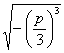,    θ=arc cos[ax3+bx2+cx+d=0]  一般三次方程

ax3+bx2+cx+d=0x=y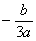y3+py+q=0

x1=y1x2=y2x3=y3x1+x2+x3=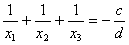,   x1x2x3=4.四次方程

[ax4+cx2+e=0]  方程

ax4+cx2+e=0

ay2+cy+e=0

x1,2,3,4=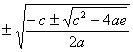[ax4+bx3+cx2+bx+a=0]  方程

ax4+bx3+cx2+bx+a=0

x1,2,3,4=y=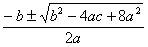[x4+bx3+cx2+dx+e=0] 一般四次方程

ax4+bx3+cx2+dx+e=0

x4+bx3+cx2+dx+e=0

x2+(b+)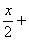(y+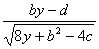)=0

x2+(b)(y)=0

8y3-4cy2+(2bd-8e)y+e(4c-b2)-d2=0

5．阿贝耳定理

五次以及更高次的代数方程没有一般的代数解法（即由方程的系数经有限次四则运算和开方运算求根的方法）.这是阿贝耳定理.